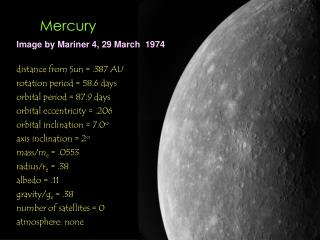DownloadDownload PresentationMercury

# Mercury

Télécharger la présentation## Mercury

- - - - - - - - - - - - - - - - - - - - - - - - - - - E N D - - - - - - - - - - - - - - - - - - - - - - - - - - -
##### Presentation Transcript

1. Image by Mariner 4, 29 March 1974 Mercury distance from Sun = .387 AU rotation period = 58.6 days orbital period = 87.9 days orbital eccentricity = .206 orbital inclination = 7.0o axis inclination = 2o mass/me = .0553 radius/re = .38 albedo = .11 gravity/ge = .38 number of satellites = 0 atmosphere: none

2. Mercury Note veins around craters, which might be lava flows induced by impact event.

3. Radar image of Mercury with Goldstone-VLA Mercury Red areas have high radar reflectivity, due either to variations in surface composition or surface roughness. The area of high radar reflectivity at the north pole (top of image) is thought to be due to significant amounts of water ice residing in permanently-shaded areas within craters in the polar region. The other two large regions of high reflectivity have never have been imaged by spacecraft, so the cause of the high reflectivity remains a mystery.

4. Mercury

5. Mercury

6. Image by Mariner 10 Venus distance from Sun = .723 AU rotation period = 243.0 days orbital period = 224.7 days orbital eccentricity = .007 orbital inclination = 7.0o axis inclination = 177.3o mass/me = .815 radius/re = .95 albedo = .65 gravity/ge = .91 number of satellites = 0 atmosphere: CO2 (97%) and N (3%)

7. Venus

8. Venus,Magellan image

9. Sif Mons on Venus

10. distance from Sun = 1 AU = 1.5x1013 cm rotation period = 1 day orbital period = 365.24 days orbital eccentricity = .017 orbital inclination = 0.0o axis inclination = 23.45o mass me = 5.98x1027 g radius re = 6.37x108 cm albedo = 0.37 gravity ge = 975 cm s-2 number of satellites = 1 atmosphere: N (80%) and O (20%) Earth, Apollo 17, December 1972

11. Earth, IR image from GOES 6 satellite and Clementine (right)

12. Sunrise over Moon, with planets

13. Moon, satellite of Earth, Apollo 17 image, December 1972

14. Earth is our home planet. Moon The uniform temperature and thermal regulation on the surface of the Earthis due to the coupling between the oceanic and atmospheric currents

15. Far Side of the Moon

16. distance from Sun = 1.52 AU rotation period = 1.029 days orbital period = 686.0 days orbital eccentricity = .093 orbital inclination = 1.8o axis inclination = 25.19o mass/me = .1074 radius/re = .533 albedo = .15 gravity/ge = .38 known satellites = 2 atmosphere: CO2 Mars, ground-based telescope

17. Mars, Mariner 4 image (1965) The large channel system is Valles Marineris

18. Mars Mars has many surface features that were formed by volcanisn, impacts, tectonics, wind, flowing water, and landslides.

19. Mars

20. Mars, Viking Orbiter

21. Phobos, satellite of Mars

22. Phobos and Deimos, satellites of Mars

23. Asteroid Gaspra

24. Asteroid Ida

25. Asteroid Ida and moon Dactyl

26. Dactyl, Moon of Ida

27. Jupiter, HST image distance from Sun = 5.20 AU rotation period = .41 days orbital period = 11.9 yrs orbital eccentricity = .048 orbital inclination = 1.3o axis inclination = 3.12o mass/me = 317.0 radius/re = .9.4 albedo = .52 gravity/ge = 2.54 known satellites = 16 atmosphere: H, He

28. Jupiter, Voyager image

29. Jupiter (detail), Voyager image

30. Io, satellite of Jupiter,Voyager image

31. Volcano Loki on Io, Voyager image

32. Europa, satellite of Jupiter

33. Europa (detail), satellite of Jupiter

34. Ganymede, satellite of Jupiter

35. Callisto, satellite of Jupiter, Voyager image

36. Image by Nordic Optical Telescope Saturn distance = 9.53 AU rotation period = .44 days orbital period = 29.5 days orbital eccentricity = .056 orbital inclination = 2.5o axis inclination = 26.73o mass/me = 95.18 radius/re = 9.4 albedo = .47 gravity/ge = 1.08 known satellites = 18 atmosphere: H, He

37. Saturn, HST

38. Saturn, Voyager image

39. Rings of Saturn,Voyager image

40. Mimas, satellite of Saturn Large impact crater is Herschel

41. Large impact crater is Telemachus Tethys, satellite of Saturn

42. Rhea, satellite of Saturn

43. Titan, satellite of Saturn

44. Iapetus, satellite of Saturn

45. Enceledus, satellite of Saturn

46. Uranus, HST WFPC2 image distance from Sun = 19.18 AU rotation period = .72 days orbital period = 84.0 yrs orbital eccentricity = .047 orbital inclination = .8o axis inclination = 97.86o mass/me = 14.53 radius/re = 4.007 albedo = .51 gravity/ge = .91 known satellites = 15 atmosphere: H and He

47. Uranus, Voyager image

48. Ariel, satellite of Uranus,Voyager image

49. Neptune, HST WFPC2 image distance from Sun = 30.06 AU rotation period = .77 days orbital period = 164.8 yrs orbital eccentricity = .009 orbital inclination = 1.8o axis inclination = 29.5o mass/me = 17.14 radius/re = 3.9 albedo = .41 gravity/ge = 1.19 known satellites = 8 atmosphere: H and He

50. Neptune, Voyager image